forsyde-atom-0.3.0.0: Shallow-embedded DSL for modeling cyber-physical systems

ForSyDe.Atom.ExB

Contents

Description

This module exports the core entities of the extended behavior layer: interfaces for atoms and common patterns of atoms. It does NOT export any implementation or instantiation of any specific behavior extension type. For an overview about atoms, layers and patterns, please refer to the ForSyDe.Atom module documentation.

IMPORTANT!!! see the naming convention rules on how to interpret, use and develop your own constructors.

Synopsis

# Atoms

class Functor b => ExB b where Source #

Class which defines the atoms for the extended behavior layer.

As its name suggests, this layer is extending the behavior of the wrapped entity/function by expanding its domains set with symbols having clearly defined semantics (e.g. special events with known responses).

The types associated with this layer can simply be describes as: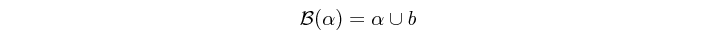where $$\alpha$$ is a base type and $$b$$ is the type extension, i.e. a set of symbols with clearly defined semantics.

Extended behavior atoms are functions of these types, defined as interfaces in the ExB type class.

Methods

extend :: a -> b a Source #

Extends a value (from a layer below) with a set of symbols with known semantics, as described by a type instantiating this class.

(/.\) :: (a -> a') -> b a -> b a' infixl 4 Source #

Basic functor operator. Lifts a function (from a layer below) into the domain of the extended behavior layer.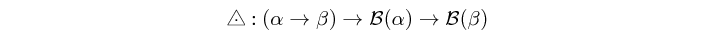(/*\) :: b (a -> a') -> b a -> b a' infixl 4 Source #

Applicative operator. Defines a resolution between two extended behavior symbols.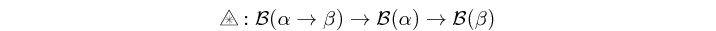(/&\) :: b Bool -> b a -> b a infixl 4 Source #

Predicate operator. Generates a defined behavior based on an extended Boolean predicate.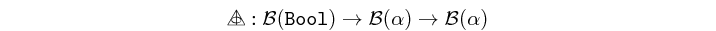(/!\) :: a -> b a -> a infixl 4 Source #

Degenerate operator. Degenerates a behavior-extended value into a non-extended one (from a layer below), based on a kernel value. Used also to throw exceptions.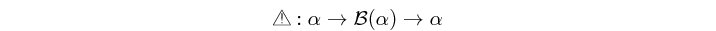#### Instances

Instances details
 Source # Implements the absent semantics of the extended behavior atoms. Instance detailsDefined in ForSyDe.Atom.ExB.Absent Methodsextend :: a -> AbstExt a Source #(/.\) :: (a -> a') -> AbstExt a -> AbstExt a' Source #(/*\) :: AbstExt (a -> a') -> AbstExt a -> AbstExt a' Source #(/!\) :: a -> AbstExt a -> a Source #

# Patterns

Arguments

 :: ExB b => (a1 -> a2 -> (a1', a2')) function on values -> b a1 first input -> b a2 second input -> (b a1', b a2') tupled outputThe res behavior pattern lifts a function on values to the extended behavior domain, and applies a resolution between two extended behavior symbols.

Constructors: res[1-8][1-4].

filter :: ExB b => b Bool -> b a -> b a Source #

Prefix name for the prefix operator /&\.

filter' :: ExB b => Bool -> a -> b a Source #

Same as filter but takes base (non-extended) values as input arguments.

degen :: ExB b => a -> b a -> a Source #

Prefix name for the degenerate operator /!\.

Arguments

 :: ExB b => (a1 -> a2 -> a1' -> a2' -> (a1, a2)) function of Y + X arguments -> a1 -> a2 -> b a1' -> b a2' -> (a1, a2)The ignoreXY pattern takes a function of Y + X arguments, Y basic inputs followed by X behavior-extended inputs. The function is first lifted and applied on the X behavior-extended arguments, and the result is then degenerated using the Y non-extended arguments as fallback. The effect is similar to "ignoring" a the result of a function evaluation if $$\in b$$.

The main application of this pattern is as extended behavior wrapper for stateful processes which do not "understand" extended behavior semantics, i.e. it simply propagates the current state $$(\in \alpha)$$ if the inputs belongs to the set of extended values $$(\in b)$$.

Constructors: ignore[1-4][1-4].